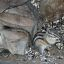ISSAC 94 - Symbolic-Numeric Nonlinear Equation Solving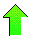Contents News View

 Results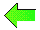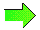The preceding algorithm has been implemented in Maple and run on various inputs including the following examples:(1)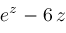for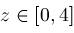(2)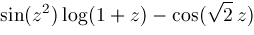forFigures 1.1-1.2 picture these two functions.

The operations of procedures Zeros and Narrow are represented in Figures 2.1-2.2.  Each right triangle represents a narrowing step by procedure Narrow.  A triangle with vertices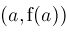,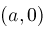,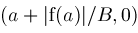represents Narrow detecting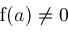and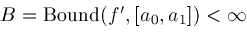allowing Narrow to eliminate subinterval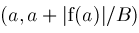as a possible location for any zeros ofThe effects of procedure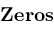can be seen wherever there is a change in the slope of the hypotenuses of the triangles or two right triangles' altitudes merge together.

Gaps on the z-axis between the triangles (most visible in Figure 2.2), represent procedures Locate and Newton taking over from procedures Zeros and Narrow to locate an isolated root of.  Closeups of this process for selected roots are shown in Figures 3.1-3.2.  Pointsin the trajectories computed by Locate and Newton are also shown.  The points converge onto the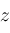-axes where the roots are located.

In Maple, we choose nsolve as the name of the main routine to resemble Maple's routine fsolve.  Procedure nsolve returns Nonlin() objects and these, like E or Pi, can be evaluated via Maple's evalf to as many decimal places as desired.  Thus,

> nsolve(E^z-6*z,z,0..4);

[Nonlin(...), Nonlin(...)]

> evalf(",30);

[.204481449339915533617757754510, 2.83314789204934214261167464234]

Each Nonlin() object records the nonlinear equation which is solved, the Newton iteration formula, a bounding interval for the root, and the two bounds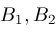needed by procedure Newton to terminate properly.